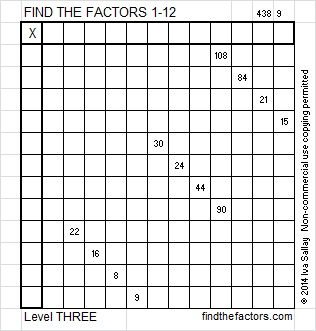# 241 and Level 3

• 241 is a prime number.
• Prime factorization: 241 is prime.
• The exponent of prime number 241 is 1. Adding 1 to that exponent we get (1 + 1) = 2. Therefore 241 has exactly 2 factors.
• Factors of 241: 1, 241
• Factor pairs: 241 = 1 x 241
• 241 has no square factors so its square root cannot be simplified. √241 ≈ 15.524How do we know that 241 is a prime number? If 241 were not a prime number, then it would be divisible by at least one prime number less than or equal to √241 ≈ 15.524. Since 241 cannot be divided evenly by 2, 3, 5, 7, 11, or 13, we know that 241 is a prime number.Print the puzzles or type the factors on this excel file: 12 Factors 2014-09-22

A Logical Approach to FIND THE FACTORS: Find the column or row with two clues and find their common factor. Write the corresponding factors in the factor column (1st column) and factor row (top row).  Because this is a level three puzzle, you have now written a factor at the top of the factor column. Continue to work from the top of the factor column to the bottom, finding factors and filling in the factor column and the factor row one cell at a time as you go.This site uses Akismet to reduce spam. Learn how your comment data is processed.